Courses

# Thin and Thick Cylinders and Spheres Civil Engineering (CE) Notes | EduRev

## Civil Engineering (CE) : Thin and Thick Cylinders and Spheres Civil Engineering (CE) Notes | EduRev

The document Thin and Thick Cylinders and Spheres Civil Engineering (CE) Notes | EduRev is a part of the Civil Engineering (CE) Course Civil Engineering SSC JE (Technical).
All you need of Civil Engineering (CE) at this link: Civil Engineering (CE)

THIN CYLINDERS

• If the thickness of the cylinder is less than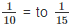of the diameter of the cylinder, it is treated as the thin cylinder.
• It is assumed that the stresses are uniformly distributed through out the thickness of the wall.
• ‘Hoop stress’ or ‘Circumferential stress’ is given by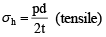• ‘Longitudinal stress’ is given by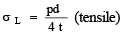• The Maximum shear stress is given by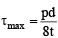• Hoop strain is given by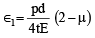• Longitudinal strain is given by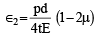• Volumetric strain is given by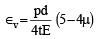Where p = internal pressure
d = diameter of cylinder
t = thickness of the cylinder
µ = Poisson's ratio

• If ‘σa’ be the permissible tensile stress for the shell material, then from strength point of view, the major principal stress ( σ ) should be less than or equal to σa Hence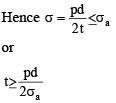THIN SPHERICAL SHELLS

• In case of thin spherical shells, longitudinal stress and circumferential stress are equal and are given by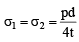(tensile in nature)

• The maximum shear stress,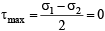• The strain in any direction is given by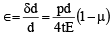• The volumetric strain is given by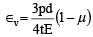CYLINDERS WITH HEMISPHERICAL ENDS
Let tc = thickness of the cylinder ts = thickness of the hemisphere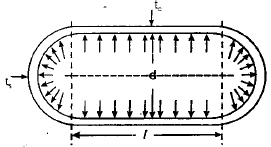• Hoop stress in cylindrical part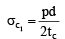• Hoop stress in hemispherical part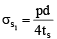• Longitudinal stress in cylindrical part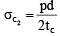• Longitudinal stress in hemispherical part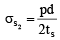• Circumferential strain in hemispherical part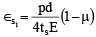• Circumferential strain in cylindrical part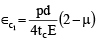• For the condition of no distortion of the junction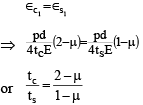• This means thickness of cylindrical part should be more than the hemispherical part.
• For the condition of same maximum stress in cylindrical and hemispherical parts,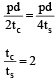THICK CYLINDRICAL SHELL

If the thickness of shell is greater thanof  its diameter then it is called thick shell.

In thick cylinders the circumferential stress no longer remains constant, but varies along the thickness and the radial pressure (px) is also not negligible.
The following three types of stresses are existing in thick cylinders :

(i) The radial pressure ‘px’ (compressive)
(ii) The hoop stress fx (tensile)
(iii) The longitudinal tensile stress po (tensile)

• The longitudinal stress may be given by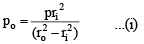• Hoop stress, is given by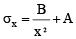• Radial pressure is given by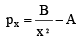Equation (ii) and (iii) are called Lame’s equation.

ro = outer radius of shell

ri = inner radius of shell A and B are Lame’s constant

Note:-

1. Longitudinal tension is uniform across the thickness.

2. Hoop tension vary form maximum at inner face to minimum at outer face Hyperbolically.

3. Radial compression varies from maximum at inner face to zero at outer face. Hyperbolically.

Offer running on EduRev: Apply code STAYHOME200 to get INR 200 off on our premium plan EduRev Infinity!

## Civil Engineering SSC JE (Technical)

109 docs|50 tests

,

,

,

,

,

,

,

,

,

,

,

,

,

,

,

,

,

,

,

,

,

;